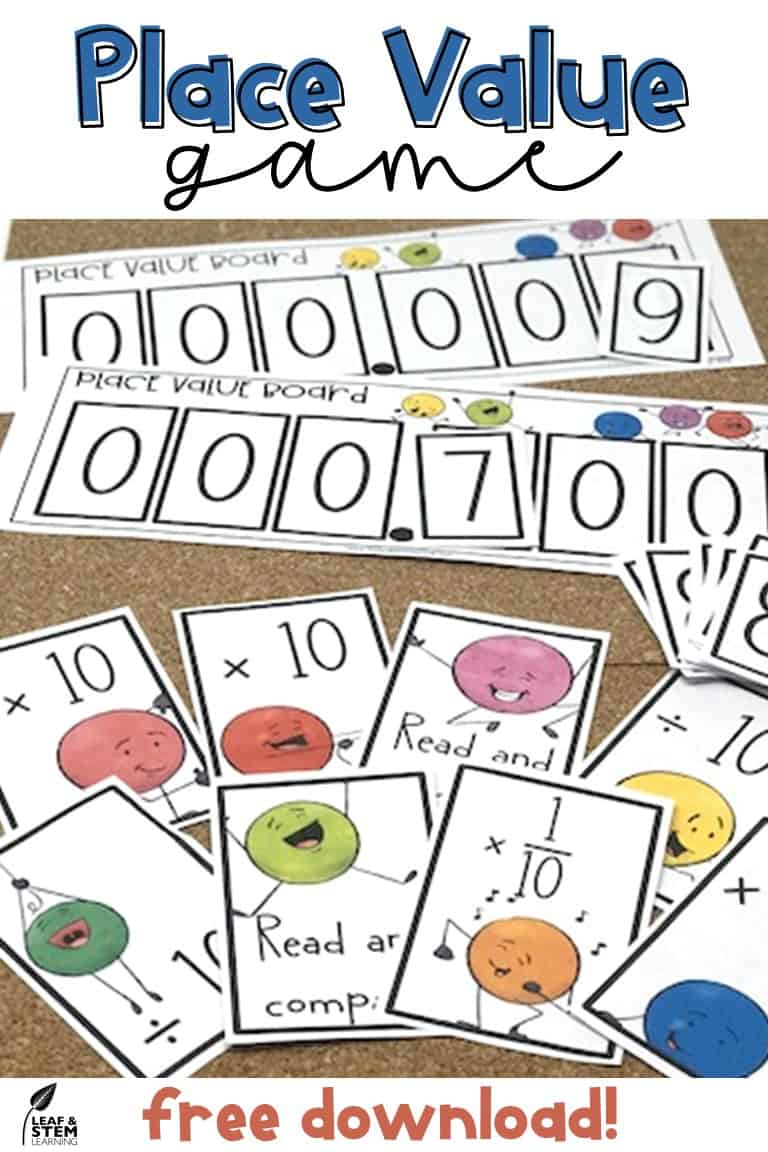Select PageBefore Texas adopted its new TEKS and before the common core became common across the US, place value instruction was generally presented differently that it is today.  Teachers all around the country have had to adjust to their new standards.

One place where the standards have changed is in the area of place value.  Rather than an emphasis on memorizing the names of each of the place value positions, the importance has shifted to knowing about the relationships between the place value positions.  Ensuring that your students deeply understand the relationship between each place value and how movement of a digit to the left or right affect the value of a digit is a must.  By fourth grade, students have to understand that moving a digit to the right represents a new value only one-tenth of the original value!  That is the beginning of fraction multiplication, a skill that was not previously introduced to students to students until middle school!  Can your students visualize a number being divided into tenths?  Base 10 blocks are a great tool to help your students process this concept.  Another fun way to master place value relationships is with games.  Games make the repetitive practice needed engaging for students!  Click on the image below to download a free place value game perfect for a wide range of ages.One question that seems to have cropped up after these changes to standards has been “what is meant by the terms expanded form or expanded notation?”

In Texas, the standards differentiate between the terms expanded form and expanded notation.  The former being a less complex skill that is mastered by third grade and the later a more complex skill mastered by around fifth grade.  In the common core standards, only the term expanded form is used, but its use in conjunction with the emphasis on base ten system fluency define it as the expanded notation concept.

So what is the difference between these two concepts?  Is there a difference, or is just a matter of semantics?  Here is what you need to know to make sure you are instructing your students to the depth and complexity expected by the standards.  Traditional expanded form would look something like a simple addition problem.  The number 734 = 700 + 30 + 4, would be an example of a whole number written in expanded form.  Expanded notation, on the other hand, is a bit more complicated.  It relies on the distributive property and knowledge of the relationships within the base ten system.  Using the same example, we would see 734 = (7 x 100) + (3 x 10) + 4, or something similar when writing an expanded notation.

Notice how, although similar, the expanded notation of the number distinctly demonstrates the relationship between the place value position of the digit and the value of that position in the number.  We also can see a variety of appearances in equivalent representations when using numbers with decimal digits.